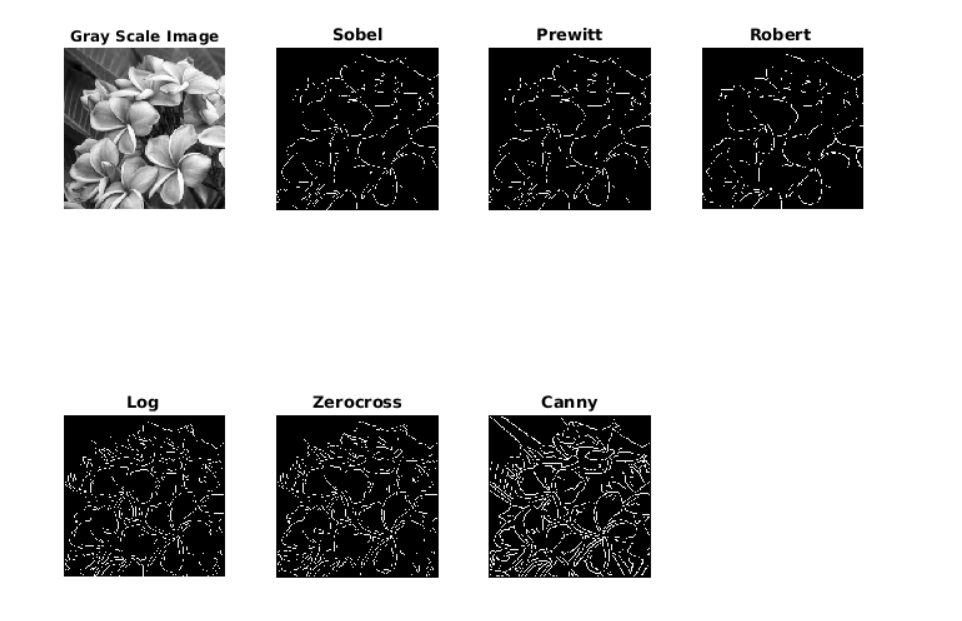# Edge detection using in-built function in MATLAB

Edge detection: In an image, an edge is a curve that follows a path of rapid change in intensity of that image. Edges are often associated with the boundaries of the object in a scene environment. Edge detection is used to identify the edges in an image to make the image processing easy. Edge detection works by detecting discontinuities in brightness. Edge detection is mainly used for image segmentation and data extraction in areas such as image processing, computer vision, and machine vision.

To find edges, you can use the in-built edge function edge(image, Edge detector) of Matlab. This in-built function looks for places in the image where the intensity changes rapidly, using one of these two criteria:

• Places where the first derivative of the intensity is larger in magnitude than some threshold value.
• Places where the second derivative of the intensity has a zero crossing.

Edge detectors provides several derivative estimators, each of which implements one of the above stated definitions. For some of these estimators, you can specify whether the operation should be sensitive to vertical edges, horizontal edges, or both. Edge estimators  returns a binary image containing 1’s where edges are found and 0’s elsewhere.
The most powerful edge-detection technique that edge provides is the Canny method. The Canny method differs from the other edge-detection methods in that it uses two different types of thresholds levels to detect strong and weak edges. The Canny edge detection method includes the weak edges in the output only if they are connected to strong edges. This method is therefore less likely to be affected by noise, and more likely to detect true weak edges.

There are many Edge detection in-built functions are available in Matlab like:

• Sobel edge detector
• Prewitt edge detector
• Robert edge detector
• Log edge detector
• Zerocross edge detector
• Canny edge detector

Edge detection using MATLAB library function.

 `% importing the imgae ` `I = rgb2gray(imread(``"flowers.jpg"``));  ` `subplot(2, 4, 1),  ` `imshow(I);  ` `title(``"Gray Scale Image"``);  ` ` `  `% Sobel Edge Detection  ` `J = edge(I, ``'Sobel'``);  ` `subplot(2, 4, 2),  ` `imshow(J);  ` `title(``"Sobel"``);  ` ` `  `% Prewitt Edge detection  ` `K = edge(I, ``'Prewitt'``);  ` `subplot(2, 4, 3),  ` `imshow(K);  ` `title(``"Prewitt"``);  ` ` `  `% Robert Edge Detection  ` `L = edge(I, ``'Roberts'``);  ` `subplot(2, 4, 4),  ` `imshow(L);  ` `title(``"Robert"``);  ` ` `  `% Log Edge Detection  ` `M = edge(I, ``'log'``);  ` `subplot(2, 4, 5),  ` `imshow(M);  ` `title(``"Log"``);  ` ` `  `% Zerocross Edge Detection  ` `M = edge(I, ``'zerocross'``);  ` `subplot(2, 4, 6),  ` `imshow(M);  ` `title(``"Zerocross"``);  ` ` `  `% Canny Edge Detection  ` `N = edge(I, ``'Canny'``);  ` `subplot(2, 4, 7),  ` `imshow(N);  ` `title(``"Canny"``);  `

Output :Attention reader! Don’t stop learning now. Get hold of all the important CS Theory concepts for SDE interviews with the CS Theory Course at a student-friendly price and become industry ready.

My Personal Notes arrow_drop_upCheck out this Author's contributed articles.

If you like GeeksforGeeks and would like to contribute, you can also write an article using contribute.geeksforgeeks.org or mail your article to contribute@geeksforgeeks.org. See your article appearing on the GeeksforGeeks main page and help other Geeks.

Please Improve this article if you find anything incorrect by clicking on the "Improve Article" button below.

Article Tags :

Be the First to upvote.

Please write to us at contribute@geeksforgeeks.org to report any issue with the above content.